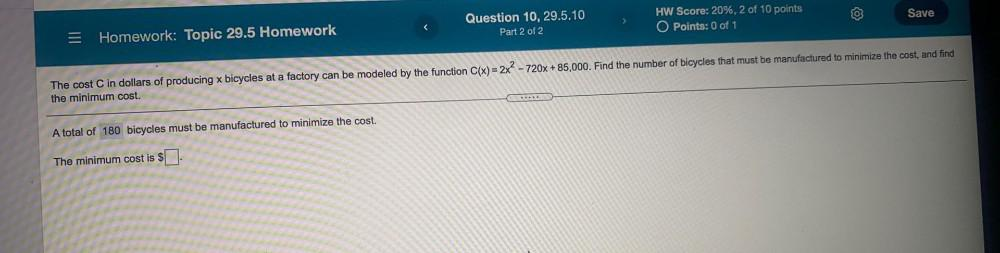Question:

# Save > Question 10, 29.5.10 Part 2 of 2 HW Score: 20%, 2 of 10 points O Points: 0 of 1 Homework: Topic 29.5 Homework The cost CSave > Question 10, 29.5.10 Part 2 of 2 HW Score: 20%, 2 of 10 points O Points: 0 of 1 Homework: Topic 29.5 Homework The cost C in dollars of producing x bicycles at a factory can be modeled by the function C(x) = 2x² - 720x +85,000. Find the number of bicycles that must be manufactured to minimize the cost, and find the minimum cost. A total of 180 bicycles must be manufactured to minimize the cost. The minimum cost is \$[